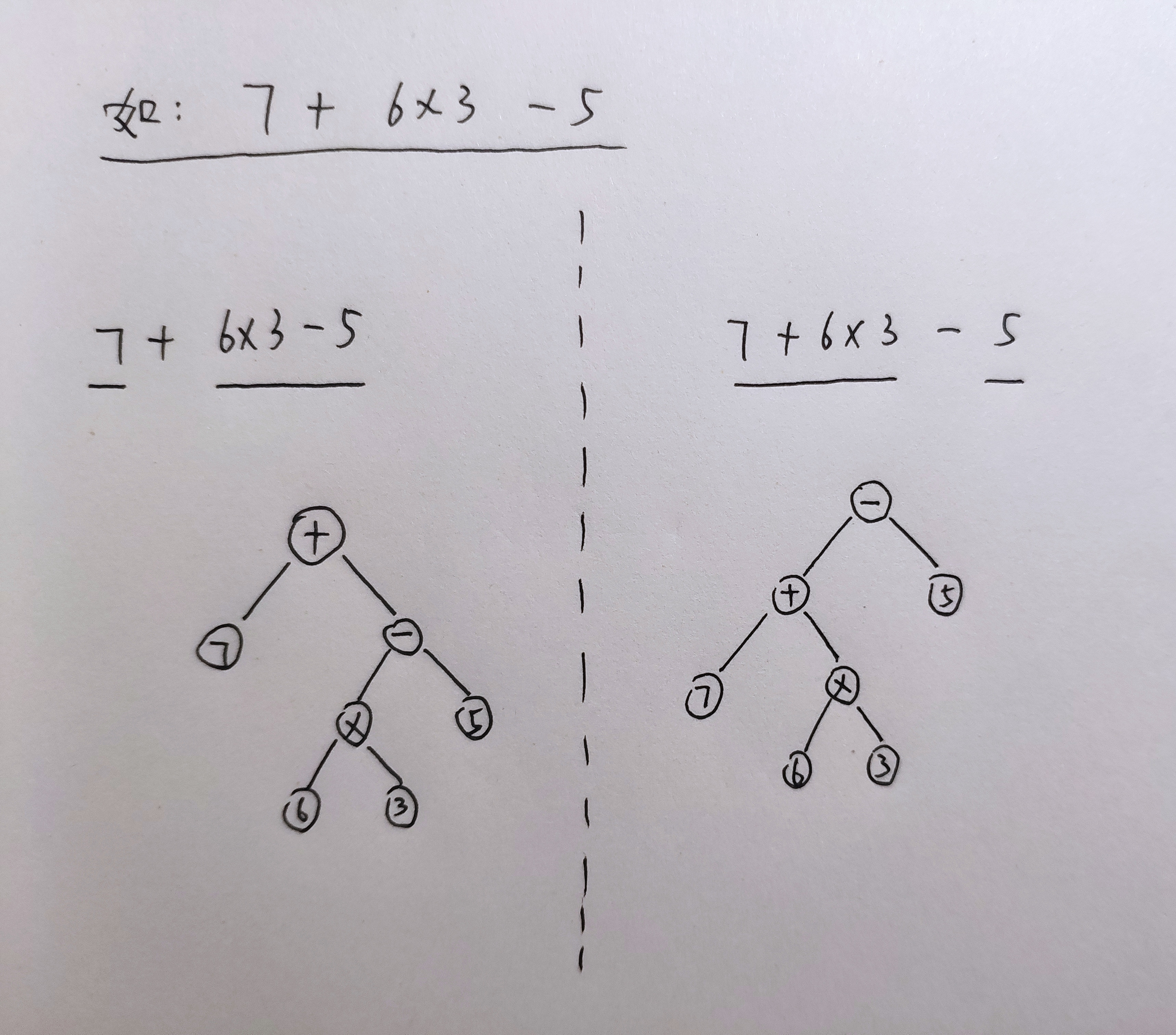# 中缀表达式转换前缀表达式and中缀表达式转换后缀表达式（利用栈）

## 写在前边## 中缀表达式转换前缀表达式

### 转换思路：

1、先对输入的字符串进行处理，分割字符串，划分优先级，将多位数的数字存入结构体看做整体，方便后续处理，为各个运算符划分优先级

2、创建两个栈，一个是结果栈，一个是运算符栈

3、从右至左扫描中缀表达式，如果是数字，就直接压入结果栈

（注意：我们先在运算符栈中压入一个‘#’  规定其优先级为最低  这样解决了开始时候的边界问题）

（注意：特殊情况，如果遇到右括号直接压入栈中，如果遇到一个左括号，那么就将栈元素弹出并压入结果栈直到遇到右括号。停止弹出，这个过程，两个括号都不加入结果栈。）

4、最后，处理完了表达式，若运算符栈中还有元素，则将元素依次弹出并压入结果栈

5、将结果栈倒序输出

### 代码实现：

//例：1-(2+3)  转换前缀是：- 1 + 2 3

//例：1+((2+3)*4)-5  转换前缀是：- + 1 * + 2 3 4 5
//例：123+((246+39)*48)-55 转换前缀是：- + 123 * + 246 39 48 55

#include<stdio.h>
#include<iostream>
#include<algorithm>
#include<string.h>
using namespace std;
const int maxn=1005;
typedef struct NODE{
string ch;
int level;
}NODE;
NODE node[maxn];
string s;
int change(){
int w1=0;
for(int i=0;i<s.size();i++){  //划分优先级
if(s[i]=='*'||s[i]=='/'){
node[w1].ch=s[i];
node[w1++].level=2;
}
else if(s[i]=='+'||s[i]=='-'){
node[w1].ch=s[i];
node[w1++].level=1;
}
else if(s[i]=='('||s[i]==')'){
node[w1].ch=s[i];
node[w1++].level=0;
}
else if(s[i]>='0'&&s[i]<='9'){ //分割字符串
int j=i;
while(s[j]>='0'&&s[j]<='9'){ j++;}
node[w1].ch=s.substr(i,j-i);
node[w1].level=100;
w1++;
i=j-1;
}
}
return w1;
}
void solove(int l){
NODE ans[maxn]; //结果栈
NODE q[maxn];  //运算符栈
int w2=0,w3=0;
q[w3].ch="#";  q[w3++].level=-1;//先压入 #
for(int i=l-1;i>=0;i--){
if(node[i].level==100){
ans[w2++]=node[i];
}
else{
if(node[i].ch==")") q[w3++]=node[i];
else if(node[i].ch=="("){
while(q[w3-1].ch!=")"){
w3--; ans[w2++]=q[w3];//压入结果栈
}
w3--;
}
else{
while(node[i].level<q[w3-1].level){ //比较优先级
w3--; ans[w2++]=q[w3];
}
q[w3++]=node[i];
}
}
}
while(w3!=1){
w3--;  ans[w2++]=q[w3];
}
for(int i=w2-1;i>=0;i--){//倒序输出
cout<<ans[i].ch<<" ";
}
return ;
}
int main(){
cin>>s;        //输入表达式
int l=change();//处理表达式
solove(l);     //转换为前缀表达式
return 0;
}


## 中缀表达式转换后缀表达式

### 转换思路：

1、先对输入的字符串进行处理，分割字符串，划分优先级，将多位数的数字存入结构体看做整体，方便后续处理，为各个运算符划分优先级

2、创建两个栈，一个是结果栈，一个是运算符栈

3、从左至右扫描中缀表达式，如果是数字，就直接压入结果栈

（注意：我们先在运算符栈中压入一个‘#’  规定其优先级为最低  这样解决了开始时候的边界问题）

（注意：特殊情况，如果遇到左括号直接压入栈中，如果遇到一个右括号，那么就将栈元素弹出并压入结果栈直到遇到左括号。停止弹出，这个过程，两个括号都不加入结果栈。）

4、最后，处理完了表达式，若运算符栈中还有元素，则将元素依次弹出并压入结果栈

5、将结果栈正序输出

### 代码实现

a/b+(c*d-e*f)/g

(2*(9+6/3-5)+4)

(a+b+c*d)/e

#include<stdio.h>
#include<iostream>
#include<algorithm>
#include<string.h>
using namespace std;
const int maxn=1005;
typedef struct NODE{
string ch;
int level;
}NODE;
NODE node[maxn];
string s;
int change(){
int w1=0;
for(int i=0;i<s.size();i++){  //划分优先级
if(s[i]=='*'||s[i]=='/'){
node[w1].ch=s[i];
node[w1++].level=2;
}
else if(s[i]=='+'||s[i]=='-'){
node[w1].ch=s[i];
node[w1++].level=1;
}
else if(s[i]=='('||s[i]==')'){
node[w1].ch=s[i];
node[w1++].level=0;
}
else if(s[i]>='0'&&s[i]<='9'||s[i]>='a'&&s[i]<='z'){ //分割字符串  //！！！分离字母
int j=i;
while(s[j]>='0'&&s[j]<='9'||s[j]>='a'&&s[j]<='z'){ j++;}
node[w1].ch=s.substr(i,j-i);
node[w1].level=100;
w1++;
i=j-1;
}
}
return w1;
}
void solove(int l){
NODE ans[maxn]; //结果栈
NODE q[maxn];  //运算符栈
int w2=0,w3=0;
q[w3].ch="#";  q[w3++].level=-1;//先压入 #
for(int i=0;i<l;i++){      //！！！从左到右扫描
if(node[i].level==100){
ans[w2++]=node[i];
}
else{
if(node[i].ch=="(") q[w3++]=node[i];   //！！！左括号
else if(node[i].ch==")"){
while(q[w3-1].ch!="("){
w3--; ans[w2++]=q[w3];//压入结果栈
}
w3--;
}
else{
while(node[i].level<=q[w3-1].level){ //比较优先级  ！！！这里加了个等号
w3--; ans[w2++]=q[w3];
}
q[w3++]=node[i];
}
}
}
while(w3!=1){
w3--;  ans[w2++]=q[w3];
}
for(int i=0;i<w2;i++){   //！！！正序输出
cout<<ans[i].ch<<" ";
}
return ;
}
int main(){
cin>>s;        //输入表达式
int l=change();//处理表达式
solove(l);     //转换为后缀表达式
return 0;
}


09-1706-05575
08-061530
05-271654
01-05130
03-201万+
09-158526
04-118891
07-083877
04-19544
05-042982
05-305万+
03-15917
11-281万+
01-224万+
03-11647
06-291588
04-27100
©️2020 CSDN 皮肤主题: 精致技术 设计师:CSDN官方博客